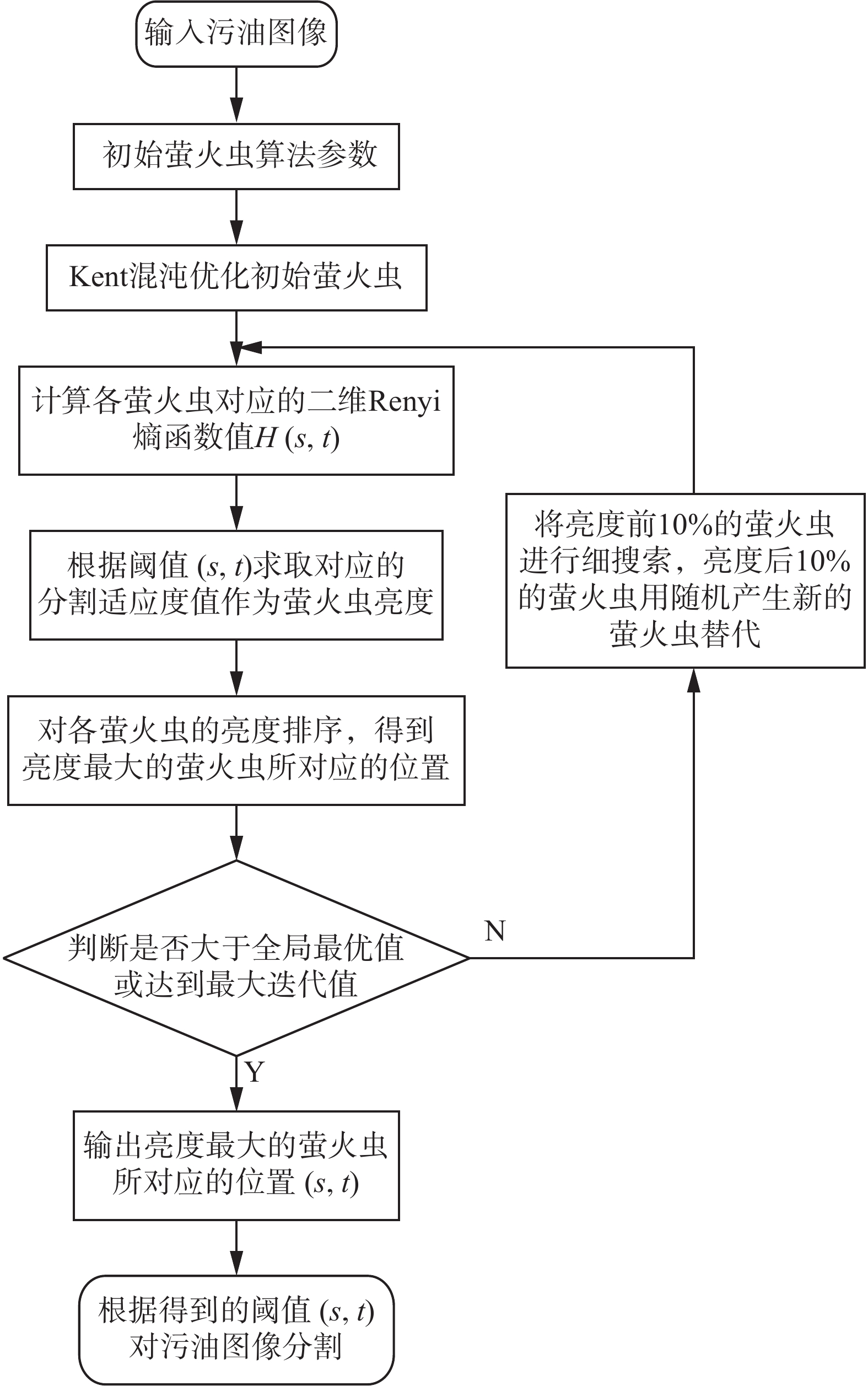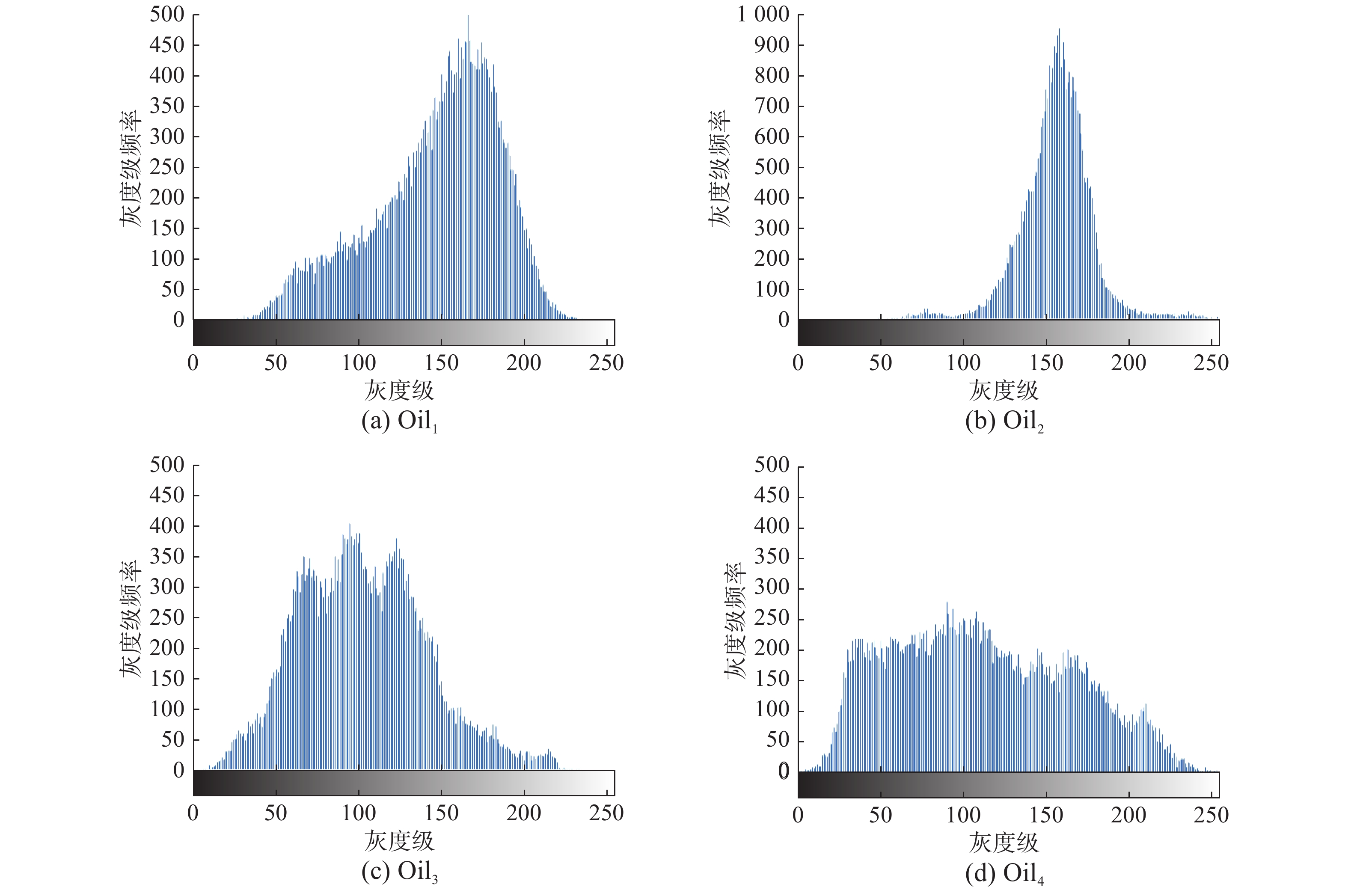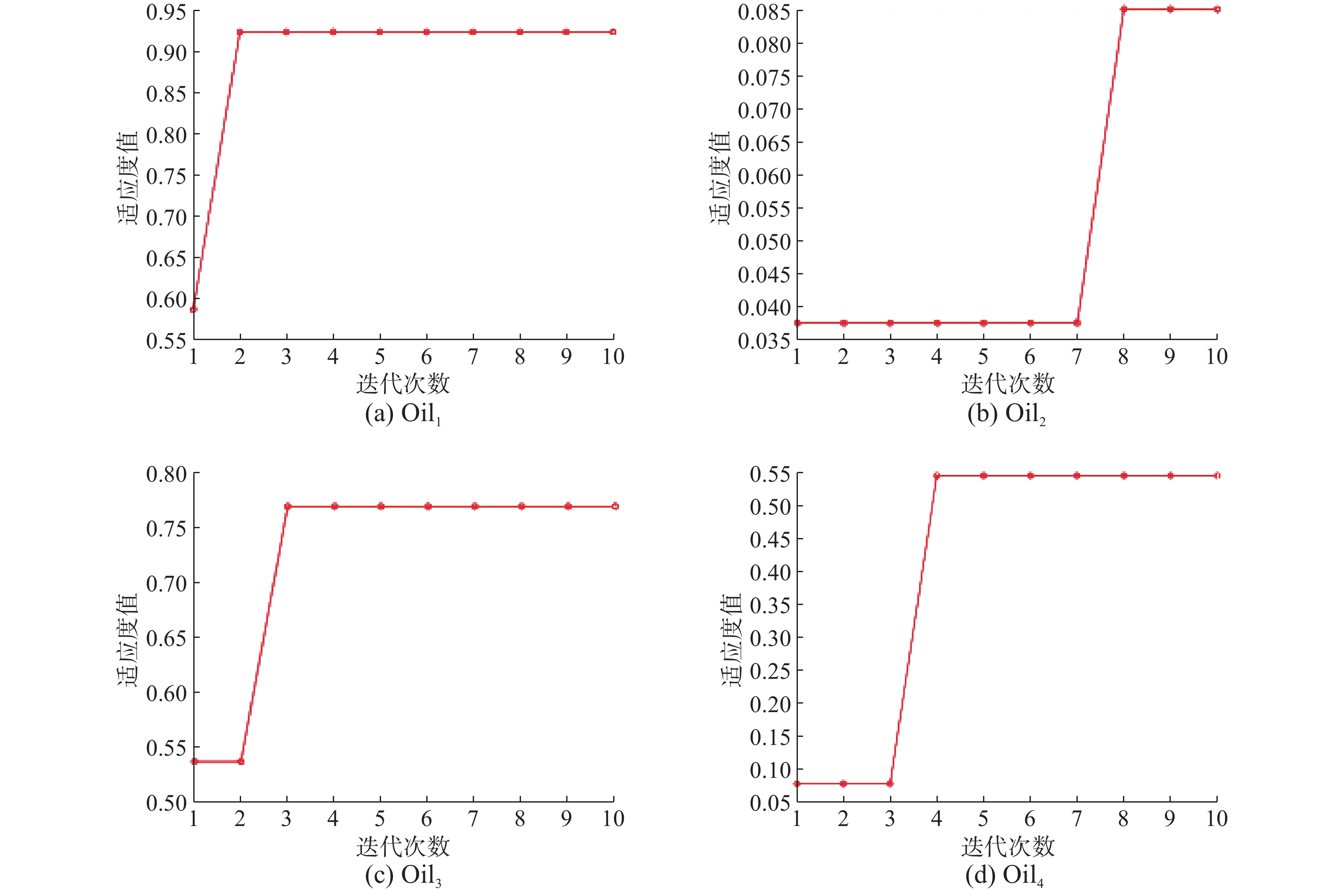﻿ 改进萤火虫优化算法的Renyi熵污油图像分割
«上一篇文章快速检索 高级检索

 智能系统学报2020, Vol. 15Issue (2): 367-373  DOI: 10.11992/tis.2018090020

### 引用本文JIA Heming, PENG Xiaoxu, XING Zhikai, et al. Renyi entropy based on improved firefly optimization algorithm for image segmentation of waste oil[J]. CAAI Transactions on Intelligent Systems, 2020, 15(2): 367-373. DOI: 10.11992/tis.201809002.### 文章历史

1. 东北林业大学 机电工程学院，黑龙江 哈尔滨 150040;
2. 大庆油田有限责任公司采油二厂，黑龙江 大庆 163000

Renyi entropy based on improved firefly optimization algorithm for image segmentation of waste oil
JIA Heming 1, PENG Xiaoxu 1, XING Zhikai 1,2, LI Jinduo 1, KANG Lifei 1
1. College of Mechanical and Electrical Engineering, Northeast Forestry University, Harbin 150040, China;
2. Daqing Oil Field Co. Oil Production Plant Two, Daqing 163000, China
Abstract: Aiming at the problem that the traditional Renyi entropy method has large image gaps and cannot be optimized according to different images when dividing dirty oil images, an improved firefly algorithm is proposed to solve the above problem by optimizing the alpha value of two-dimensional Renyi entropy segmentation algorithm. First, we analyze the characteristics of an acquired oil image and the necessity of segmenting a dirty oil picture; second, aiming at the problems of low optimization precision and slow convergence speed in the later stage, the firefly algorithm is improved to make the initial position of the firefly chaos optimization processing results reach the global optimum, and then Renyi entropy image segmentation algorithm based on the improvement of the firefly algorithm is applied to the experiments of threshold value segmentation of the waste oil image. Finally, the algorithm proposed in this paper is used to collect oil image segmentation in experiments, and the results are compared with the 2D Renyi entropy segmentation and the particle swarm optimization (PSO) Renyi entropy segmentation method. The experimental results illustrate that the proposed algorithm can effectively segment the waste oil area and quickly achieve accurate processing of complex images.
Key words: image processing of waste oil    threshold segmentation    firefly algorithm    two-dimensional Renyi entropy    chaos optimization    multi-objective optimization    fitness learning    global optimization

1 改进的萤火虫优化算法 1.1 萤火虫算法

1)萤火虫相对亮度为

 $I = {I_0} \times {{\rm e}^{ - \gamma {r_{ij}}}}$

2)萤火虫的吸引度为

 $\beta = {\beta _0} \times {{\rm e}^{ - \gamma {r_{ij}}}}$

3)萤火虫i向萤火虫j移动的位置更新公式为

 ${x_i} = {x_j} + \beta ({x_i} - {x_j}) + {\alpha _1} \times ({\rm rand} - 0.5)$

1.2 混沌优化策略

 {x_{n + 1}} = \left\{ {\begin{aligned} & {\frac{{{x_n}}}{\beta },0 < {x_n} < \beta } \\ & {\frac{{(1 - {x_n})}}{{(1 - \beta )}},\beta < {x_n} < 1} \end{aligned}} \right. (1)

 $\left\{ {\begin{array}{*{20}{c}} {{x_{\min ,j}} = \max \{ {x_{\min ,j}},{x_{g,j}} - \rho ({x_{\max ,j}} - {x_{\min ,j}})\} } \\ {{x_{\max ,j}} = \min \{ {x_{\max ,j}},{x_{g,j}} + \rho ({x_{\max ,j}} - {x_{\min ,j}})\} } \end{array}} \right.$ (2)

1.3 基于改进萤火虫的α选取算法

 $\widetilde {{f_k}} = \frac{1}{{{N_k}}}\sum\limits_{i \in {R_k}} {{f_i}}$ (3)
 $\sigma _k^2 = \frac{1}{{{N_k}}}\sum\limits_{i \in {R_k}} {{{({f_i} - \widetilde {{f_k}})}^2}}$ (4)
 ${U_I} = 1 - \dfrac{2}{N}\sum\limits_{{R_k} \in I} {\dfrac{{\displaystyle\sum\limits_{i \in {R_k}} {{{({f_i} - \frac{1}{{{N_k}}}\displaystyle\sum\limits_{i \in {R_k}} {{f_i}} )}^2}} }}{{{{({{\max }_{i \in {R_k}}}{f_i} - {{\min }_{i \in {R_k}}}{f_i})}^2}}}}$ (5)

 $\widehat \alpha = {\rm Arg}\max {U_I}(t(\alpha )),\alpha > 0$ (6)

2 基于改进萤火虫算法的二维Renyi熵阈值分割方法

1)萤火虫吸引度修正公式：

 $\beta = {\beta _0} \times {{\rm e}^{ - \gamma {r_i}}}$ (7)

2)萤火虫位置修正公式：

 $x_k^i = x_k^i + \beta ({g_{\rm bestk}} - x_k^i) + {\alpha _1} \times ({\rm rand} - 0.5)$ (8)

 ${{H}}(1) = \frac{1}{{1 - \alpha }}\ln \sum\limits_{i = 0}^s {\sum\limits_{j = 0}^t {{{(\frac{{{P_{ij}}}}{{{P_1}(s,t)}})}^\alpha }} }$
 ${{H}}(2) = \frac{1}{{1 - \alpha }}\ln \sum\limits_{i = s + 1}^{L - 1} {\sum\limits_{j = s + 1}^{L - 1} {{{(\frac{{{P_{ij}}}}{{{P_2}(s,t)}})}^\alpha }} }$ (9)

 $H(s,t) = H(1) + H(2)$ (10)

 $({s^*},{t^*}) = \mathop {\arg \max }\limits_{1 \leqslant s,t \leqslant L - 1} \{ H(s,t)\}$

1)产生一个 $(0,1)$ 之间的随机数，代入式(2)应用混沌优化策略获得初始萤火虫。

2)对每个萤火虫的适应度值进行计算。为了求出图像的最优阈值t，利用式(9)、(10)计算第i个萤火虫的Renyi熵( $\alpha = {x_i}$ )；运用式(5)求出其适应度值。通过以上的方法，经过多次运算，求出全部适应度值。

3)模拟萤火虫优化过程并更新萤火虫的位置。根据式(7)计算所有萤火虫的适应度值与全局最优值之间的吸引度；根据式(8)更新萤火虫位置。

4)具有最初10%亮度的萤火虫被优化为用于混沌搜索的优异粒子，具有最后10%亮度的萤火虫被随机产生的新萤火虫取代。

5)若迭代到终止阈值T或者最大迭代次数M，则停止进行迭代；否则，转入步骤2)。Download: 图 1 改进萤火虫算法的二维Renyi熵阈值分割算法流程图 Fig. 1 The flow chart of the two-dimensional Renyi entropy threshold segmentation algorithm is improved
3 污油图像分割实验与分析Download: 图 2 图像分割对比处理结果 Fig. 2 Image segmentation and comparison processing resultsDownload: 图 3 4幅原始图像的灰度直方图 Fig. 3 The grayscale histogram of the four original images表 1 算法的运行时间和信噪比值 Tab.1 Algorithm running time and PSNR value

PSNR准则的相关表达式为

 ${\rm PSNR} = 20\log \left(\frac{{255}}{{\rm RMSE}}\right)(\rm dB)$ (11)
 ${\rm RMSE} = \sqrt {\frac{{\displaystyle\sum\limits_{i = 1}^M {\displaystyle\sum\limits_{j = 1}^N {{{(I(i,j) - \hat I(i,j))}^2}} } }}{{MN}}}$ (12)Download: 图 4 适应度函数迭代优化学习曲线 Fig. 4 The fitness function iteratively optimizes the learning curve

4 结束语

  王翔宇, 王跃, 鲍蕊, 等. 基于巡检方案事件检出概率的长距管线无人机总体设计[J]. 航空学报, 2016, 37(1): 193-206. WANG Xiangyu, WANG Yue, BAO Rui, et al. Conceptual design for long-distance pipeline patrolling UVA based on detection probability of patrolling scheme[J]. Acta aeronautica et astronautica sinica, 2016, 37(1): 193-206. (0)  张彪, 杜景涛, 许海银. 基于物联网技术的数字油田三维管线智能巡检系统[J]. 智慧建筑与城市, 2017(9): 50-53. ZHANG Biao, DU Jingtao, XU Haiyin. Intelligent patrol inspection system of digital oilfield based on IOT technology[J]. Intelligent building and city, 2017(9): 50-53. (0)  陈雨, 蒋连江. 石油管线智能巡检系统应用与研究[J]. 石油石化管线与技术, 2009, 3(3): 120-123. (0)  马英辉, 吴一全. 利用混沌布谷鸟优化的二维Renyi灰度熵图像阈值选取[J]. 智能系统学报, 2018, 13(1): 152-158. MA Yinghui, WU Yiquan. Two-dimensional Renyi-gray-entropy image threshold selection based on chaotic cuckoo search optimization[J]. CAAL transactions on intelligent systems, 2018, 13(1): 152-158. (0)  陈超, 宣士斌, 雷红轩. 基于狼群算法与二维最大熵的图像分割[J]. 计算机工程, 2018, 44(1): 233-237. CHEN Chao, XUAN Shibin, LEI Hongxuan. Image segmentation based on wolf pack algorithm and 2D maximum entropy[J]. Computer engineering, 2018, 44(1): 233-237. DOI:10.3969/j.issn.1000-3428.2018.01.039 (0)  YANG Xinshe. Nature-inspired metaheuristic algorithms[M]. Frome, UK: Luniver Press, 2008: 180-186. (0)  刘佳昆, 周永权. 一种最大最小萤光素值人工萤火虫算法[J]. 计算机应用研究, 2011, 28(10): 3662-3664. LIU Jiakun, ZHOU Yongquan. Glowworm swarm optimization algorithm based on max-min luciferin[J]. Application research of computers, 2011, 28(10): 3662-3664. DOI:10.3969/j.issn.1001-3695.2011.10.015 (0)  DOS SANTOS COELHO L, DE ANDRADE BERNERT D L, MARIANI V C. A chaotic firefly algorithm applied to reliability-redundancy optimization[C]//Proceedings of 2011 IEEE Congress of Evolutionary Computation. New Orleans, LA, USA, 2011: 517–521. (0)  冯艳红, 刘建芹, 贺毅朝. 基于混沌理论的动态种群萤火虫算法[J]. 计算机应用, 2013, 33(3): 796-799, 805. FENG Yanhong, LIU Jianqin, HE Yichao. Chaos-based dynamic population firefly algorithm[J]. Journal of computer applications, 2013, 33(3): 796-799, 805. DOI:10.3969/j.issn.1001-3695.2013.03.039 (0)  KULKARNI R V, VENAYAGAMOORTHY G K. Bio-inspired algorithms for autonomous deployment and localization of sensor nodes[J]. IEEE transactions on systems, man, and cybernetics, part C (applications and reviews), 2010, 40(6): 663-675. DOI:10.1109/TSMCC.2010.2049649 (0)  刘毅, 黄兵, 孙怀江, 等. 利用视觉显著性与图割的图像分割算法[J]. 计算机辅助设计与图形学学报, 2013, 25(3): 402-409. LIU Yi, HUANG Bing, SUN Huaijiang, et al. Image segmentation based on visual saliency and graph cuts[J]. Journal of computer-aided design & computer graphics, 2013, 25(3): 402-409. DOI:10.3969/j.issn.1003-9775.2013.03.016 (0)  刘洪彬, 常发亮. 权重系数自适应光流法运动目标检测[J]. 光学精密工程, 2016, 24(2): 460-468. LIU Hongbin, CHANG Faliang. Moving object detection by optical flow method based on adaptive weight coefficient[J]. Optics and precision engineering, 2016, 24(2): 460-468. (0)  HORNG M H. Vector quantization using the firefly algorithm for image compression[J]. Expert systems with applications, 2012, 39(1): 1078-1091. DOI:10.1016/j.eswa.2011.07.108 (0)  马英辉, 吴一全. 基于改进的指数交叉熵和萤火虫群优化的工业CT图像分割[J]. 图学学报, 2017, 38(1): 57-62. MA Yinghui, WU Yiquan. Segmentation of industrial CT image based on improved exponential cross entropy and glow-worm swarm optimization[J]. Journal of graphics, 2017, 38(1): 57-62. DOI:10.11996/JG.j.2095-302X.2017010057 (0)  周晨航, 田力威, 赵宏伟. 基于改进萤火虫算法的二维Otsu图像分割法[J]. 沈阳大学学报(自然科学版), 2016, 28(1): 45-50. ZHOU Chenhang, TIAN Liwei, ZHAO Hongwei. Image thresholding segmentation with 2-D Otsu based on improved firefly algorithm[J]. Journal of Shenyang University (Natural Science), 2016, 28(1): 45-50. DOI:10.3969/j.issn.2095-5456.2016.01.009 (0)